×

Search anything:

# Gaussian Naive Bayes

#### Machine Learning (ML)Indian Technical Authorship Contest starts on 1st July 2023. Stay tuned.

Gaussian Naive Bayes is a variant of Naive Bayes that follows Gaussian normal distribution and supports continuous data. We have explored the idea behind Gaussian Naive Bayes along with an example.

Before going into it, we shall go through a brief overview of Naive Bayes.

Naive Bayes are a group of supervised machine learning classification algorithms based on the Bayes theorem. It is a simple classification technique, but has high functionality. They find use when the dimensionality of the inputs is high. Complex classification problems can also be implemented by using Naive Bayes Classifier.

# Bayes Theorem

Bayes Theorem can be used to calculate conditional probability. Being a powerful tool in the study of probability, it is also applied in Machine Learning.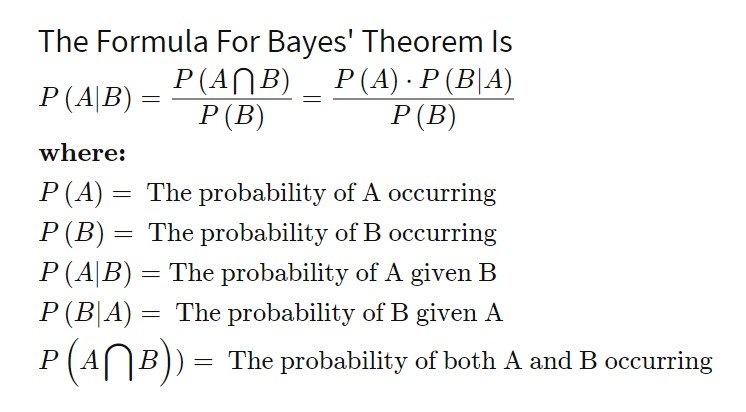Bayes Theorem has widespread usage in variety of domains.

# Naive Bayes Classifier

Naive Bayes Classifiers are based on the Bayes Theorem. One assumption taken is the strong independence assumptions between the features. These classifiers assume that the value of a particular feature is independent of the value of any other feature. In a supervised learning situation, Naive Bayes Classifiers are trained very efficiently. Naive Bayed classifiers need a small training data to estimate the parameters needed for classification. Naive Bayes Classifiers have simple design and implementation and they can applied to many real life situations.

# Gaussian Naive Bayes

When working with continuous data, an assumption often taken is that the continuous values associated with each class are distributed according to a normal (or Gaussian) distribution. The likelihood of the features is assumed to be-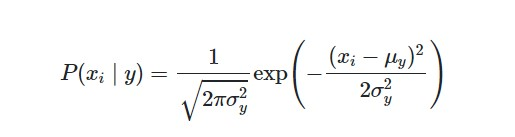Sometimes assume variance

• is independent of Y (i.e., σi),
• or independent of Xi (i.e., σk)
• or both (i.e., σ)

Gaussian Naive Bayes supports continuous valued features and models each as conforming to a Gaussian (normal) distribution.

An approach to create a simple model is to assume that the data is described by a Gaussian distribution with no co-variance (independent dimensions) between dimensions. This model can be fit by simply finding the mean and standard deviation of the points within each label, which is all what is needed to define such a distribution.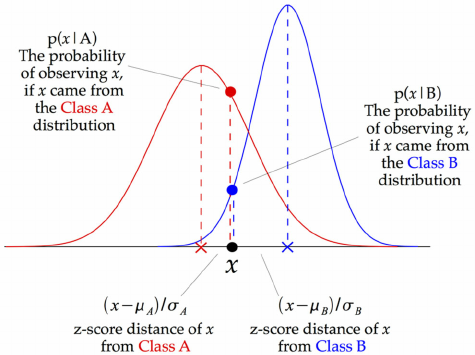The above illustration indicates how a Gaussian Naive Bayes (GNB) classifier works. At every data point, the z-score distance between that point and each class-mean is calculated, namely the distance from the class mean divided by the standard deviation of that class.

Thus, we see that the Gaussian Naive Bayes has a slightly different approach and can be used efficiently.

Let's do a practical implementation of the Gaussian Naive Bayes using Python and Scikit Learn. The data we will be working on for the exercise looks like this-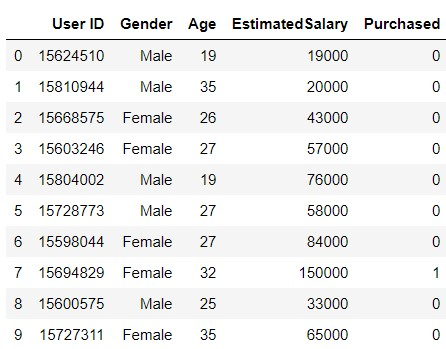This is a database of various people based on their gender, age and estimated salary. The target is whether the person buys a SUV or not.
If the "Purchased" column has value "1", then it means that the person has bought the SUV, and if the value is "0", then it means that the person has not bought the SUV.

Lets start now.
Here we import the necessary libraries.

``````#Importing the libraries

import numpy as np
import matplotlib.pyplot as mtp
import pandas as pd
``````

Now we import the data.

``````# Importing the data
x = dataset.iloc[:, [2, 3]].values
y = dataset.iloc[:, 4].values
``````

Let us take a look at the data.

``````#taking a look at the data

``````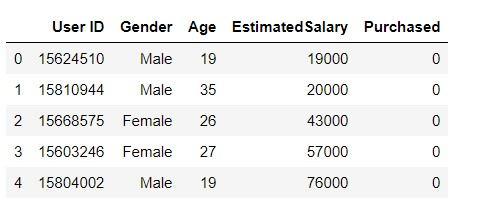Now we do the step of splitting data into test and train sets.

``````#splitting the data into training and testing sets

from sklearn.model_selection import train_test_split
x_train, x_test, y_train, y_test = train_test_split(x, y, test_size = 0.25, random_state = 5)
``````

``````# Feature Scaling

from sklearn.preprocessing import StandardScaler
sc = StandardScaler()
x_train = sc.fit_transform(x_train)
x_test = sc.transform(x_test)
``````

Applying the classifier model.

``````# Fitting Naive Bayes to the Training set

from sklearn.naive_bayes import GaussianNB
classifier = GaussianNB()
classifier.fit(x_train, y_train)
``````
``````# Predicting the Test set results

y_pred = classifier.predict(x_test)
``````
``````#Accuracy score

from sklearn.metrics import accuracy_score

accuracy_score(y_test, y_pred)
``````

It gives a value of 0.89, hence a 89% accuracy.

Next we try to find the confusion matrix.

A confusion matrix is a performance measurement method for Machine learning classification. It helps you to the know the performance of the classification model on a set of test data for that the true values and false are known. It helps us find out, how mant times our model has given correct or wrong output and of what type. Hence, it is a very important tool for evaluating classification models.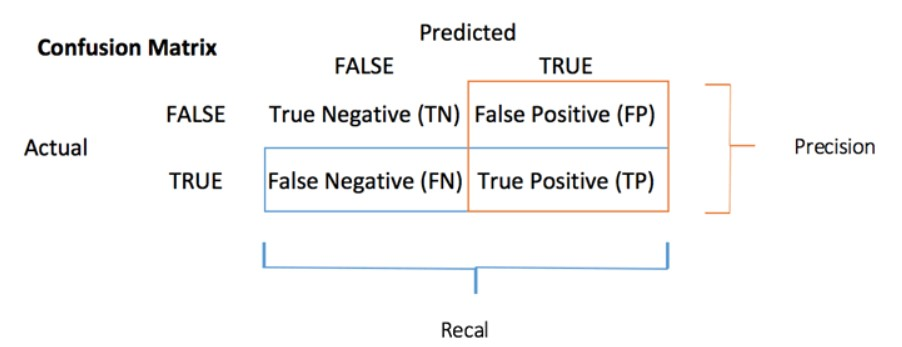So, there are net 4 types of outcomes possible.

• TP: True Positive: Predicted values correctly predicted as actual positive
• FP: False Positive: Predicted values incorrectly predicted an actual positive. i.e., Negative values predicted as positive
• FN: False Negative: Positive values predicted as negative
• TN: True Negative: Predicted values correctly predicted as an actual negative

We can compute the accuracy test from the confusion matrix: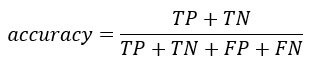``````#Confusion Matrix

from sklearn.metrics import confusion_matrix
cm = confusion_matrix(y_test, y_pred)

print(cm)
``````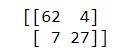Our result comes as given above. We can see that,

• TP: 27
• FP: 4
• FN: 7
• TN: 62

Accuracy= (89/100)= 0.89

Hence an 89% accuracy.

After visualising the results, we see.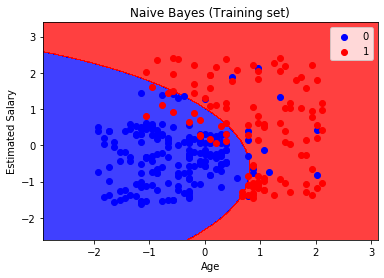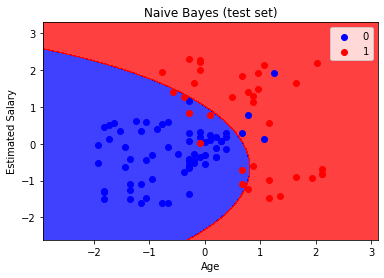Hence, we can say that Gaussian Naive Bayes Classifier works well and can be used for a variety of Classification Problems.